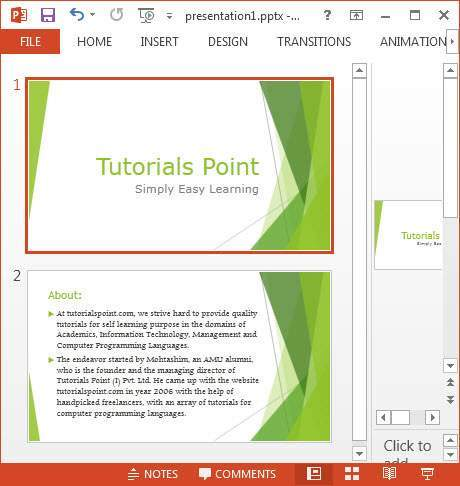# Apache POI PPT - PPT to Image

You can convert a presentation to an image file. The following program shows how to go about it.

```import java.awt.Color;
import java.awt.Dimension;
import java.awt.Graphics2D;
import java.awt.geom.Rectangle2D;
import java.awt.image.BufferedImage;
import java.io.File;
import java.io.FileInputStream;
import java.io.FileOutputStream;
import java.io.IOException;

import org.apache.poi.xslf.usermodel.XMLSlideShow;
import org.apache.poi.xslf.usermodel.XSLFSlide;

public class PptToImage {

public static void main(String args[]) throws IOException {

//creating an empty presentation
File file=new File("pptToImage.pptx");
XMLSlideShow ppt = new XMLSlideShow(new FileInputStream(file));

//getting the dimensions and size of the slide
Dimension pgsize = ppt.getPageSize();
XSLFSlide[] slide = ppt.getSlides();

BufferedImage img = null;

for (int i = 0; i < slide.length; i++) {
img = new BufferedImage(pgsize.width, pgsize.height,BufferedImage.TYPE_INT_RGB);
Graphics2D graphics = img.createGraphics();

//clear the drawing area
graphics.setPaint(Color.white);
graphics.fill(new Rectangle2D.Float(0, 0, pgsize.width, pgsize.height));

//render
slide[i].draw(graphics);

}

//creating an image file as output
FileOutputStream out = new FileOutputStream("ppt_image.png");
javax.imageio.ImageIO.write(img, "png", out);
ppt.write(out);

System.out.println("Image successfully created");
out.close();
}
}
```

Save the above Java code as PpttoPNG.java, and then compile and execute it from the command prompt as follows −

```\$javac PpttoPNG.java
\$java PpttoPNG
```

It will compile and execute to generate the following output −

```Image created successfully
```

The following snapshot shows the presentation that is given as input −Given below is the snapshot of the image created at the specified location.